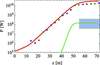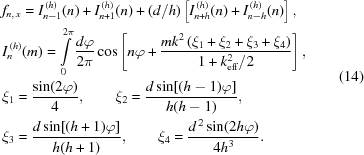JOURNAL OFSYNCHROTRONRADIATION
ISSN: 1600-5775
Volume 26| Part 2| March 2019| Pages 605-606

## Phenomenological and numerical analysis of power evolution and bunching in single-pass X-ray FELs. Erratum

aDepartment of Theoretical Physics, Faculty of Physics, M. V. Lomonosov Moscow State University, Moscow 119991, Russian Federation
*Correspondence e-mail: zhukovsk@physics.msu.ru

(Received 8 December 2018; accepted 4 February 2019; online 28 February 2019)

A correction is made to the paper by Zhukovsky & Kalitenko (2019). [J. Synchrotron Rad. 26, 159–169].

In our previous work (Zhukovsky & Kalitenko, 2019) we modelled and commented on an LCLS experiment, stating that the third harmonic was not registered in it. We followed Emma et al. (2010) and realised that we were mistaken. Indeed, a later study of the third and second harmonics in this experiment was reported by Ratner et al. (2011). The radiation of the third harmonic was registered in the hard X-ray LCLS experiment.

Our phenomenological modelling of the harmonic power of 1.5 Å free-electron laser (FEL) radiation in the LCLS experiment for γ = 26600, σ = 1 × 10−4, x,y = 0.4 µm rad, λu = 3 cm, k = 3.5 was presented in Fig. 5 of Zhukovsky & Kalitenko (2019). Now we add to it the comparison with the experimentally measured data from Ratner et al. (2011) and Emma et al. (2010). The fundamental harmonic measurements correspond to the coloured dots, following the data of Emma et al. (2010). The experimentally allowed range for the third harmonic is denoted by the shadowed area, following the data of Ratner et al. (2011). The third harmonic in our phenomenological simulation — the green line — fits in the middle of the range [see Fig. 1below, which complements Fig. 5 in our earlier work (Zhukovsky & Kalitenko, 2019)].Figure 1 Phenomenological modelling of the harmonic power in the 1.5 Å FEL radiation LCLS experiment for γ = 26600, σ∊ = 1 × 10−4, ∊x,y = 0.4 µm rad, λu = 3 cm, k = 3.5. Harmonics in the phenomenological model (solid lines): n = 1, red; n = 3, green. The shadowed area denotes the range of experimental values for the third harmonics, reported by Ratner et al. (2011).

A perfect fit of the predicted values in the experimentally measured range further confirms the validity of our approach and modelling. Some higher than measured saturated power for the fundamental tone is explained by the fact that the phenomenological model gives the peak power for the fundamental tone and does not reproduce its oscillations in the saturated regime. The second harmonic was not registered in the hard X-ray band; it was registered only in the soft X-ray band and was ∼20–50 times weaker than the third harmonic: 0.04–0.1% of the fundamental tone power (Ratner et al., 2011). The saturated power of the second harmonic, estimated in the framework of the phenomenological approach, appeared lower than the initial power of the fundamental tone. Thus it is not present in Fig. 1; it also agrees with the results of Ratner et al. (2011).

We apologize for the misprinted formula (14)in the original paper (Zhukovsky & Kalitenko, 2019). The correct version follows:Further study will be presented in a dedicated forthcoming publication.

### ReferencesJOURNAL OFSYNCHROTRONRADIATION